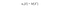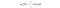# Probability theory: Moments, Variance, Standard deviation

This is part of the course “Probability Theory and Statistics for Programmers”.

In the previous article, we had a look at characteristics, that shows the position of a random variable on the numerical axis. But there is also a number of characteristics which describe certain distribution properties — Moments. Most of the time we will deal with two types of moments — initial and central.

The initial moment of the s-th order of a discrete random variable X is a sum of the form:the initial moment of the s-th order of a discrete random variable

For continuous random variable we have:the initial moment of the s-th order of a continuous random variable X

As you can see that the first initial moment is expected value. Also for the initial moment, we can join two previous formulas in one.the initial moment of the s-th order of a random variable X

Let’s see how distribution function will change according to the expected value.

Before we approach central moment we introduce the central random variable. For random variable X with expected value m. Central random variable is deviation of a random variable from its expected value:

Thus, the central moment of the s-th random variable X is the expected value of the s-th degree of the corresponding central random variable. For a discrete random variable:

And for continuous random variable:

A very important characteristic is the second central moment — Variance. Variance is characteristic of dispersion, scatter of values of a random variable near its expected value.

Let’s see how distribution function will change according to the expected value.

For a visual characteristic of dispersion, it is more convenient to use a quantity whose dimension coincides with the dimension of the random variable. To do this, a square root is extracted from the dispersion. The value obtained is called the standard deviation. In practice, you will see a standard deviation more often then variance.

Expected value, variance and standard deviation — the most commonly used characteristics of a random variable. They characterize the most important features of the distribution: its position and degree of dispersion. For a more detailed description of the distribution, higher-order moments are used. But they are out of the scope of this article.

Next part ->

Reach the next level of focus and productivity with increaser.org.

Written by